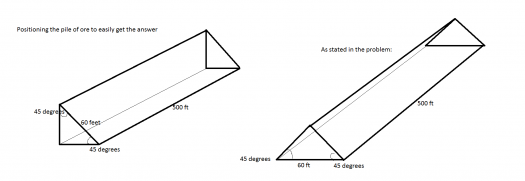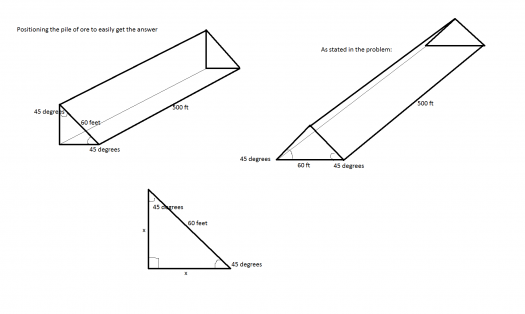# SOLID MENSURATION KERN BLAND p64

2 posts / 0 new
Ben Josef CerilloSOLID MENSURATION KERN BLAND p64

paki solve

volume of a pile of ore with a 60x500 ft rectangular base with sides inclines 45degrees to the horizontal

fitzmerl duronTo get the volume of the pile of ore, which looks like this:We need to know the formula for the volume of the figure above. The formula applicable would be:

$$V = Bh$$

where $V$ is volume of the figure, $B$ is the area of base and $h$ is the height of the figure.

We notice that we don't know the area of the end bases of this pile of ore. We need to get it by using the formula to get the area of the triangle.Getting the other sides of the pile of ore:

$$\cos 45^o = \frac{x}{60}$$ $$x = 30\sqrt{2} \space ft$$

Now getting the area of the ends of this pile of ore:

$$Area = \frac{1}{2}bh$$ $$Area = \frac{1}{2}(30\sqrt{2} \space feet)(30\sqrt{2} \space feet)$$ $$Area = 900 \space ft^2$$

Now we can get the volume of this pile of ore:

$$V= Bh$$ $$V = (900 \space ft^2)(500 \space ft)$$ $$V = 450000 \space ft^3$$

Therefore the volume of your pile of ore is $\color{green}{450000 \space ft^3}$

Alternate solutions are encouraged...heheheheh

Subscribe to MATHalino on